Courses

Test: Basic Concepts : PN Junction

10 Questions MCQ Test Analog Electronics | Test: Basic Concepts : PN Junction

Description
Attempt Test: Basic Concepts : PN Junction | 10 questions in 10 minutes | Mock test for Electrical Engineering (EE) preparation | Free important questions MCQ to study Analog Electronics for Electrical Engineering (EE) Exam | Download free PDF with solutions
QUESTION: 1

If 120 C of charge passes through an electric conductor in 60 sec, the current in the conductor is:

Solution:

i = dQ/dt = 120/60 = 2A.

QUESTION: 2

A solid copper sphere, 10 cm in diameter is deprived of 1020 electrons by a charging scheme. The charge on the sphere is:

Solution:

n = 1020
Q = ne = e * 1020 = 16.02 C.
The charge on the sphere will be positive.

QUESTION: 3

The energy required to move 120 coulomb through 3 V is:

Solution:

W = QV = 360 J.

QUESTION: 4

A lightning bolt carrying 30,000 A lasts for 50 microseconds. If the lightning strikes an airplane flying at 20,000 feet, what is the charge deposited on the plane?

Solution: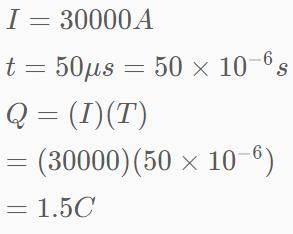QUESTION: 5

Consider the circuit graph shown in figure below. Each branch of circuit graph represent a circuit element. The value of voltage V1 is​: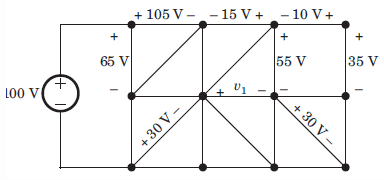Solution:
• 100 = 65 + V2 ⇒ V2 = 35 V
• V3 – 30 = V2 ⇒ V3 = 65 V
• 105 – V3 + V4 – 65 = 0 ⇒ V4 = 25 V
• V4 + 15 – 55 + V1 = 0 ⇒ V1 = 15 V.
QUESTION: 6

What is the value of Req = ?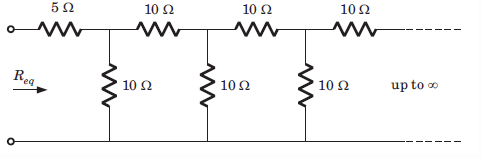Solution:
• Req – 5 = 10(Req + 5)/(10 + 5 +Req).
• Solving for Req we have
Req = 11.18 ohm.
QUESTION: 7

The superposition theorem requires as many circuits to be solved as there are:

Solution:
QUESTION: 8

Twelve 6 resistor are used as edge to form a cube. The resistance between two diagonally opposite corner of the cube is: (in ohm)

Solution: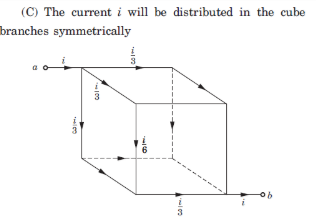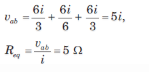QUESTION: 9

The energy required to charge a 10 µF capacitor to 100 V is:

Solution:

Energy provided is equal to 0.5 CV= 0.5x10-6 x 100 x 100 = 0.05 J

QUESTION: 10

A capacitor is charged by a constant current of 2 mA and results in a voltage increase of 12 V in a 10 sec interval. The value of capacitance is ________.

Solution:
• The capacitor current is given as i=C*(dv/dt), where dv/dt is the derivative of voltage, dt=t2-t1 given as 10 sec and dv is the change in voltage which is given as 12 V.
• So, we have C = i / (dv/dt)
C = 2mA/(12/10) = 2mA/(1.2).
• Hence C = 1.67mFUse Code STAYHOME200 and get INR 200 additional OFF Use Coupon Code

Track your progress, build streaks, highlight & save important lessons and more!

Similar ContentRelated tests